## List of Symbols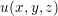Displacement field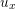Displacement field in the x-direction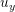Displacement field in the y-directionDisplacement field in the z-direction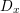Difference between displacement fields in the x-direction,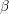Congruent skew angles in the xy plane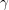,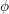Congruent skew angles in the yz plane,Congruent skew angles in the xz planeNormal strain in the i-direction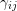Engineering shear strain in the ij plane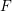External force applied in a solid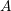Surface areaStress tensorStrain tensor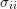Normal stress in the i-directionShear stress in the ij plane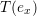Traction in the x-direction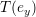Traction in the y-direction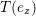Traction in the z-directionLinear mapping between stress and strain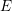Young’s modulusPoisson ratioShear stressShear modulus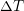Temperature variation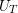Total strain energy per unit of area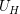Hydrostatic strain energy per unit of area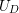Distortion strain energy per unit of area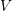Sub-space with infinity dimension of continuous with piecewise continuous derivativesAn element in V space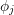FEM basis function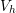Discrete sub-space of V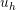A sample function in the sub-space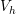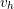A second sample function in the sub-space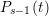Lagrangian polynomial in the BDF method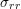Normal stress in r direction of a cylindrical coordinate system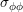Normal stress indirection of a cylindrical coordinate system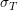Thermal stress due to temperature variation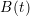Bézier polygon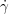Strain rate in the low temperature dislocation glide model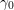Pre-exponential factor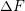Activation energy of an obstacle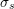Shear stress in the low temperature dislocation glide model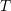Temperature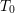Initial temperature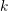Boltzmann constant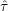Shear stress required to trigger dislocations movement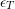Thermal strain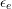Elastic strain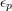Plastic strain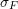Stress in the film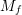Biaxial modulusSchmid factor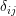Dirac deltaTimeStrain rate tensor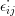Components of the strain rate tensor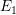System free energy per unit of area in Hoffman’s model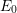Free surface energy per unit of area ot island’s top in Hoffman’s model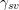Free surface energy per unit of area of the island’s lateral surface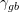Energy per unit of area spent in the formation of a grain boundary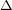Critical distance between two islands in Hoffman’s model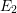Free energy per unit of area after coalescence in Hoffman’s model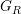Strain energy release rate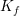Stress intensity factor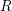Island radius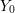Height of island coalescence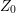Maximum displacement of the island surface due to coalescence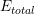Total energy in Seel’s model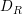Deposition rate